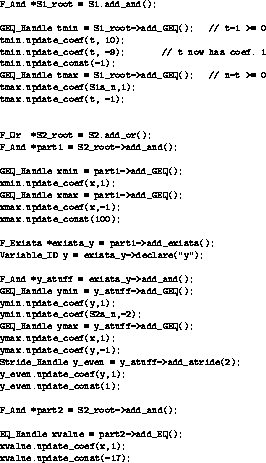Next: 4.4 Building atomic constraints Up: 4 Building New Relations Previous: 4.2 Building formulas

# 4.3 Referring to variables

An object of class Var_Decl represents all uses of a variable in a particular relation. Var_Decl s should never be created explicitly by application programs; they are created implicitly by member functions of various library classes. We will usually use Variable_ID s, which are pointers to Var_Decl s, to refer to such objects. To create constraints on a particular variable, it is necessary to determine the Variable_ID of that variable in the relation to which we want to add the constraints.

We now explain how to obtain Variable_ID s for various types of variables:

Input, output, and set variables
The Relation member functions input_var(int n) , output_var(int n) , and set_var(int n) produce Variable_ID s for thevariable in the appropriate tuple. It is illegal request an input or output variable of a set, or to request a set variable from a relation.

Quantified Variables
The member function Variable_ID F_Declaration::declare creates a new Var_Decl and add it to the list of variables associated with the F_Declaration node that it is called on. It returns the Variable_ID for the newly created variable in the current relation. If a String is specified as an argument to the member function then that String is recorded as the name of that variable, otherwise the variable remains unnamed.

Scalar Global Variables
The variables we have seen so far have all been local to a single relation. Global variables, on the other hand, may be shared between relations. A global variable is created by creating an object of a class derived from Global_Var_Decl . The class Free_Var_Decl is an example of such a class, and is used for the global variables in the code in our examples (e.g., the creation of the global variables n and m

in Figure 4.1)). New subclasses of Global_Var_Decl can be created to keep track of any additional information that applications may want to record about global variables. The member function Variable_ID Relation::get_local(const Global_Var_Decl *)

is used to return the Variable_ID of a scalar global variable in a particular relation. In Figure 4.1, the Variable_ID Rs_n is used to identify the global variable n in the relation R .

Global Variables Representing Uninterpreted Function Symbol
As was the case for scalar global variables, global variables representing uninterpreted function symbol are created by creating objects of a class derived from Global_Var_Decl . Once again, class Free_Var_Decl is an example of such a class, but this time we need to use a Free_Var_Decl constructor that allows us to specify an arity, as shown by the creation of f in Figure 4.1. The enumeration Argument_Tuple , which contains the values Input_Tuple , Output_Tuple , and Set_Tuple , is used to specify the tuple to which an uninterpreted function symbol is to be applied. Since an uninterpreted function symbol can be applied to both the input and output tuple of a relation, two Var_Decls per relation may be necessary: one for the function applied to the input tuple and the other for the function applied to the output tuple. The member function Relation::get_local(const Global_Var_Decl *, Argument_Tuple) . is used to return the Variable_ID of one of these Var_Decls

depending on which Argument_Tuple is requested. For example, we use this function to get the Variable_ID 's for Rs_f_in and Rs_f_out for the global variable f in Figure 4.1.

There are a few things you can find out about a variable given its Variable_ID :

String Var_Decl::base_name
The name of the variable without primes.

Var_Type Var_Decl::type()
Returns the type of the variable; one of { Input_Var , Output_Var , Set_Var , Global_Var , Forall_Var , Exists_Var , Wildcard_Var } (a Wildcard_Var is equivalent to an existentially quantified variable).

int Var_Decl::get_position()
If the variable is an input, output, or set variable, returns its position in the tuple.

Global_Var_ID Var_Decl::get_global_var()
If a variable corresponds to a global variable, returns its Global_Variable_ID .

Argument_Tuple Var_Decl::function_of()
If a variable corresponds to a global variable, returns the argument to which it is being applied.

Note that there is no way to find out which relation a Variable_ID is associated with, and our implementation is free to either share Variable_ID s between relations or not (i.e., the result of S1.set_var(1) == S2.set_var(1) is not defined).Figure 4.2: Example, Part 2: Adding Constraints to S1 and S2Figure 4.3: Example, Part 3: Adding Constraints to R

Figures 4.3 and 4.3 show many uses of formula building functions, as well as the creation of many equality and inequality constraints (see below). The creation of the variable y in the middle of Figure 4.3 shows the use of F_Declaration::declare(Const_String) .Next: 4.4 Building atomic constraints Up: 4 Building New Relations Previous: 4.2 Building formulas

omega@cs.umd.edu

Web Accessibility